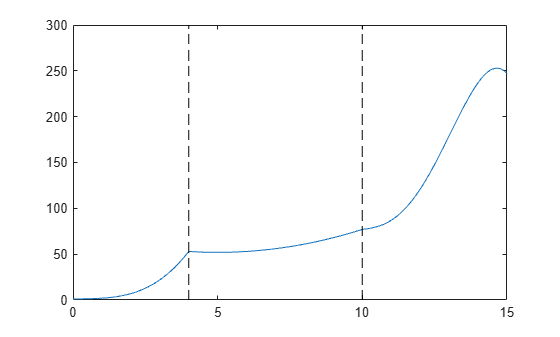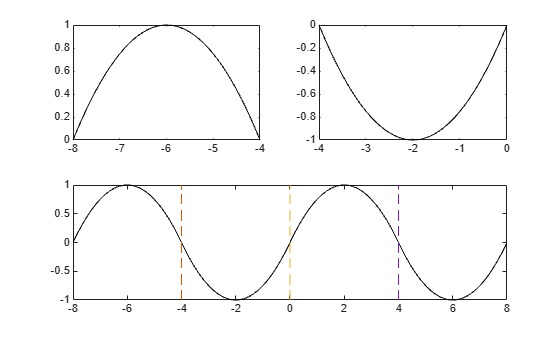Main Content

# mkpp

Make piecewise polynomial

## Syntax

``pp = mkpp(breaks,coefs)``
``pp = mkpp(breaks,coefs,d)``

## Description

example

````pp = mkpp(breaks,coefs)` builds a piecewise polynomial `pp` from its breaks and coefficients. Use `ppval` to evaluate the piecewise polynomial at specific points, or `unmkpp` to extract details about the piecewise polynomial.```
````pp = mkpp(breaks,coefs,d)` specifies that the piecewise polynomial is vector-valued, such that the value of each of its coefficients is a vector of length `d`.```

## Examples

collapse all

Create a piecewise polynomial that has a cubic polynomial in the interval [0,4], a quadratic polynomial in the interval [4,10], and a quartic polynomial in the interval [10,15].

```breaks = [0 4 10 15]; coefs = [0 1 -1 1 1; 0 0 1 -2 53; -1 6 1 4 77]; pp = mkpp(breaks,coefs)```
```pp = struct with fields: form: 'pp' breaks: [0 4 10 15] coefs: [3x5 double] pieces: 3 order: 5 dim: 1 ```

Evaluate the piecewise polynomial at many points in the interval [0,15] and plot the results. Plot vertical dashed lines at the break points where the polynomials meet.

```xq = 0:0.01:15; plot(xq,ppval(pp,xq)) line([4 4],ylim,'LineStyle','--','Color','k') line([10 10],ylim,'LineStyle','--','Color','k')```Create and plot a piecewise polynomial with four intervals that alternate between two quadratic polynomials.

The first two subplots show a quadratic polynomial and its negation shifted to the intervals [-8,-4] and [-4,0]. The polynomial is

`$1-{\left(\frac{x}{2}-1\right)}^{2}=\frac{-{x}^{2}}{4}+x.$`

The third subplot shows a piecewise polynomial constructed by alternating these two quadratic pieces over four intervals. Vertical lines are added to show the points where the polynomials meet.

```subplot(2,2,1) cc = [-1/4 1 0]; pp1 = mkpp([-8 -4],cc); xx1 = -8:0.1:-4; plot(xx1,ppval(pp1,xx1),'k-') subplot(2,2,2) pp2 = mkpp([-4 0],-cc); xx2 = -4:0.1:0; plot(xx2,ppval(pp2,xx2),'k-') subplot(2,1,2) pp = mkpp([-8 -4 0 4 8],[cc;-cc;cc;-cc]); xx = -8:0.1:8; plot(xx,ppval(pp,xx),'k-') hold on line([-4 -4],ylim,'LineStyle','--') line([0 0],ylim,'LineStyle','--') line([4 4],ylim,'LineStyle','--') hold off```## Input Arguments

collapse all

Break points, specified as a vector of length `L+1` with strictly increasing elements that represent the start and end of each of `L` intervals.

Data Types: `single` | `double`

Polynomial coefficients, specified as an `L`-by-`k` matrix with the ith row `coefs(i,:)` containing the local coefficients of an order `k` polynomial on the ith interval, `[breaks(i), breaks(i+1)]`. In other words, the polynomial is ```coefs(i,1)*(X-breaks(i))^(k-1) + coefs(i,2)*(X-breaks(i))^(k-2) + ... + coefs(i,k-1)*(X-breaks(i)) + coefs(i,k)```.

Data Types: `single` | `double`

Dimension, specified as a scalar or vector of integers. Specify `d` to signify that the piecewise polynomial has coefficient values of size `d`.

Data Types: `single` | `double`

## Output Arguments

collapse all

Piecewise polynomial, returned as a structure. Use this structure with the `ppval` function to evaluate the piecewise polynomial at one or more query points. The structure has these fields.

FieldDescription
`form`

`'pp'` for piecewise polynomial

`breaks`

Vector of length `L+1` with strictly increasing elements that represent the start and end of each of `L` intervals

`coefs`

`L`-by-`k` matrix with each row `coefs(i,:)` containing the local coefficients of an order `k` polynomial on the ith interval, `[breaks(i),breaks(i+1)]`

`pieces`

Number of pieces, `L`

`order`

Order of the polynomials

`dim`

Dimensionality of target

Since the polynomial coefficients in `coefs` are local coefficients for each interval, you must subtract the lower endpoint of the corresponding knot interval to use the coefficients in a conventional polynomial equation. In other words, for the coefficients `[a,b,c,d]` on the interval `[x1,x2]`, the corresponding polynomial is

`$f\left(x\right)=a{\left(x-{x}_{1}\right)}^{3}+b{\left(x-{x}_{1}\right)}^{2}+c\left(x-{x}_{1}\right)+d\text{\hspace{0.17em}}.$`

## See Also

Introduced before R2006a

Download ebook